Courses

# Solutions of Refraction of Light (Page No- 219) - Physics By Lakhmir Singh, Class 10 Class 10 Notes | EduRev

## Class 10 : Solutions of Refraction of Light (Page No- 219) - Physics By Lakhmir Singh, Class 10 Class 10 Notes | EduRev

The document Solutions of Refraction of Light (Page No- 219) - Physics By Lakhmir Singh, Class 10 Class 10 Notes | EduRev is a part of the Class 10 Course Lakhmir Singh & Manjit Kaur Solutions: Class 10 Science.
All you need of Class 10 at this link: Class 10

Page No:219

Question 1: If a ray of light goes from a rarer medium to a denser medium, will it bend towards the normal or away from it ?

Solution : It will bend towards the normal.

Question 2: If a ray of light goes from a denser medium to a rarer medium, will it bend towards the normal or away from the normal ?

Solution : It will bend away from the normal.

Question 3: A beam of light travelling in a rectangular glass slab emerges into air. Draw a ray-diagram indicating the change in its path.

Solution : A ray of light travelling from the glass slabs and emerges into the air.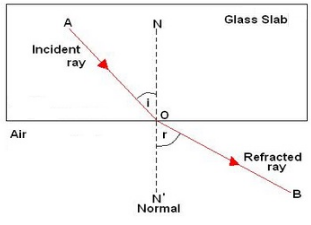Question 4: A beam of light travelling in air is incident on water. Draw a ray-diagram indicating the change in its path in water.

Solution :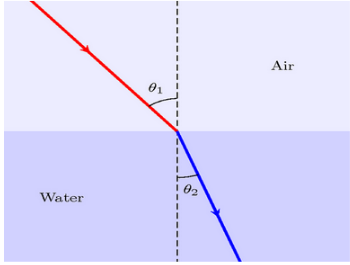Question 5: A ray of light travelling in water emerges into air. Draw a ray-diagram indicating the change in its path.

Solution : A ray of light travelling through water to air.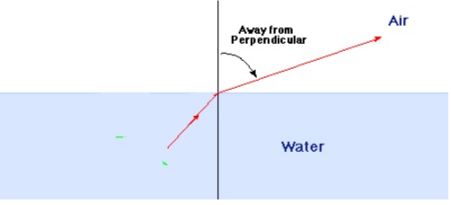Question 6: A ray of light travelling in air is incident on a parallel-sided glass slab (or rectangular glass slab). Draw a ray-diagram indicating the change in its path in glass.

Solution : A ray of light incidence on parallel sided glass slab.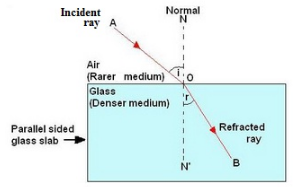Question 7: A ray of light travelling in glass emerges into air. State whether it will bend towards the normal or away from the normal.

Solution : The ray of light will bend away from the normal.

Question 8: A ray of light travelling in air enters obliquely into water. Does the ray of light bend towards the normal or away from the normal ? Why ?

Solution : The ray of light bends towards the normal. This is because water is an optically denser medium than air.

Question 9: A ray of light goes from water into air. Will it bend towards the normal or away from the normal ?

Solution : It will bend away from the normal.

Question 10: State two effects caused by the refraction of light.

Solution : Two effects caused by refraction of light are:
– a pool of water appears to be less deep than it actually is.
– an object placed under water appears to be raised.

Question 11: Name the phenomenon due to which a swimming pool appears less deep than it really is.

Solution : This is due to refraction of light.

Question 12: When a ray of light passes from air into glass, is the angle of refraction greater than or less than the angle of incidence ?

Solution : Angle of refraction is less than the angle of incidence.

Page No:220

Question 13: A ray of light passes from air into a block of glass. Does it bend towards the normal or away from it ?

Solution : A ray of light travelling from air to glass block, will bend towards the normal.

Question 14: As light rays pass from water into glass, are they refracted towards the normal or away from the normal ?

Solution : A ray of light travelling from water into glass will bend towards the normal.

Question 15: In which material do you think light rays travel faster-glass or air ?

Solution : Light rays travel faster in air.

Question 16: Which phenomenon of light makes the water to appear shallower than it really is ?

Solution : Refraction of light.

Question 17: State whether the following statement is true or false :

Refraction occurs because light slows down in denser materials.

Solution : True.

Question 18: Why does a ray of light bend when it travels from one medium to another ?

Solution : A ray of light bends when it travels from one medium to another due to the change in the speed of light.

Question 19: Fill in the following blanks with suitable words :
(a) Light travelling along a normal is…………
(b) Light bends when it passes from water into air. We say that it is……………

Solution : (a) not.
(b) refracted.

Question 20: What is meant by ‘refraction of light’ ? Draw a labelled ray diagram to show the refraction of light.

Solution : The change in direction of light when it passes from one medium to another obliquely, is called refraction of light.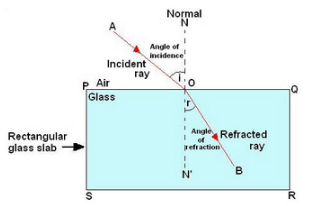Question 21: A ray of light travelling in air is incident on a rectangular glass block and emerges out into the air from the opposite face. Draw a labelled ray diagram to show the complete path of this ray of light. Mark the two points where the refraction of light takes place. What can you say about the final direction of ray of light ?

Solution :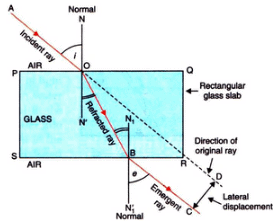The final direction of the ray of light is same as the incident direction.

Question 22: Draw a labelled ray diagram to show how a ray of light is refracted when it passes :
(a) from air into an optically denser medium.
(b) from an optically denser medium into air.

Solution : (a) Ray of light travelling from air into an optically denser medium.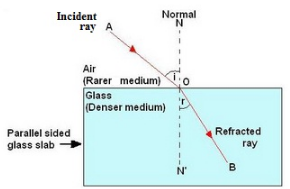(b) Ray of light travelling from an optically denser medium into air.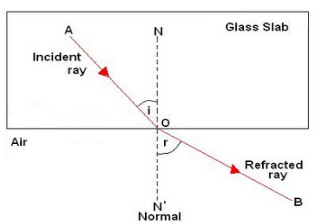Question 23: The diagram given alongside shows a ray of light entering a rectangular block of glass.
(a) Copy the diagram and draw the normal at the point of entry.
(b) Draw the approximate path of the ray of light through the glass block and out of the other side.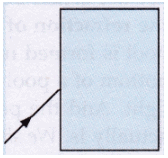Solution :

(a)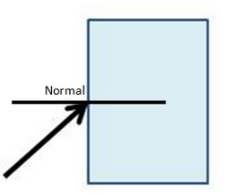(b)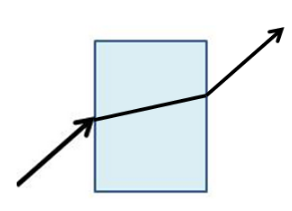Question 24: What is meant by the ‘angle of incidence’ and the ‘angle of refraction’ for a ray of light ? Draw a labelled ray diagram to show the angle of incidence and the angle of refraction for a refracted ray of light.

Solution : The angle between the incident ray and normal at the point of incidence is called angle of incidence. The angle between the refracted ray and normal at the point of refraction is called angle of refraction.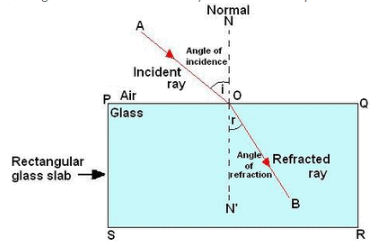Question 25: Light travels more quickly through water than through glass.
(a) Which is optically denser : water or glass ?
(b) If a ray of light passes from glass into water, which way will it bend : towards the normal or away from the normal ?

Solution : (a) Glass is optically denser than the water.
(b) The ray will bend away from the normal.

Question 26: Draw a labelled ray diagram to show how a ray of light passes through a parallel sided glass block :
(a) if it hits the glass block at 90° (that is, perpendicular to the glass block)
(b) if it hits the glass block at an angle other than 90° (that is, obliquely to the glass block).

Solution : (a) If ray of light hits the block at 90°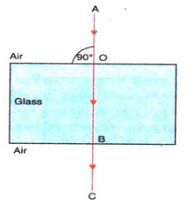(a) If ray of light hits the block other than the 90°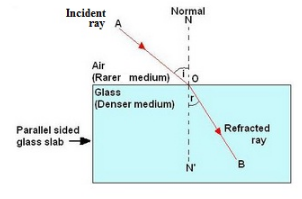Question 27: When a light ray passes from air into glass, what happens to its speed ? Draw a diagram to show which way the ray of light bends.

Solution :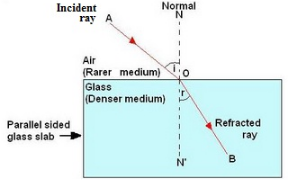The ray of light bends towards the normal.

Question 28: (a) Explain why, a stick half immersed in water appears to be bent at the surface. Draw a labelled diagram
(b) A coin in a glass tumbler appears to rise as the glass tumbler is slowly filled with water. Name the phenomenon responsible for this effect.

Solution : (a) The apparent bending of the stick is due to the refraction of light when it passes from water into air.
A ray of light OC coming from O passes from water into air and gets refracted away from normal (along CX). Another ray OD gets refracted along DY. The two refracted rays CX and DY, when produced backwards, appear to meet at point I. Thus, I is the virtual image of the end O of the stick. So, the stick appears to be bent as shown below.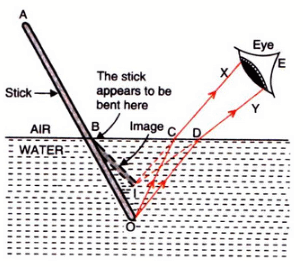(b) This phenomenon is due to the refraction of light as it comes out from water into air?

Question 29: (a) With the help of a labelled diagram, explain why a tank full of water appears less deep than it actually is.
(b) Name the phenomenon due to which a pencil partly immersed in water and held obliquely appears to be bent at the water surface.

Solution : (a) If we look into a tank of water, it appears to be less deep than it really is. This is due to the refraction of light which takes place when light rays pass from the tank of water into air. When we look into the tank, we do not see the actual bottom of the tank, we see a virtual image of the bottom of the pool which is formed by the refraction of light coming from the water into the air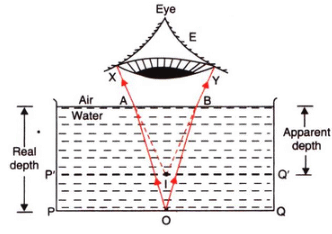(b) Refraction of light.

Question 30: (a) With the help of a diagram, show how when light falls obliquely on the side of a rectangular glass slab,
the emergent ray is parallel to the incident ray.
(b) Show the lateral displacement of the ray on the diagram.
(c) State two factors on which the lateral displacement of the emergent ray depends.

Solution :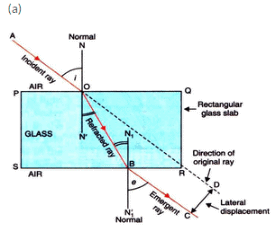(b) The lateral displacement is shown in the above diagram.
(c) Factors on which the lateral displacement depends are:
(i) Angle of incidence
(ii) Thickness of glass slab
(iii) Refractive index of glass slab

Question 31: Explain with the help of a labelled ray diagram, why a pencil partly immersed in water appears to be bent at the water surface. State whether the bending of pencil will increase or decrease if water is replaced by another liquid which is optically more dense than water.

Solution : A pencil placed in water appears to be bent because of refraction of light. The refraction causes an apparent shift in the position of the part of the pencil within the water.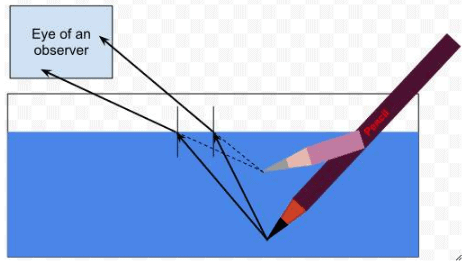If water is replaced by another liquid which is optically more dense than water, then the bending of the pencil will increase. This is because the optically denser medium will cause more refraction of light rays

Question 39: Which of the following diagrams shows the ray of light refracted correctly ?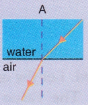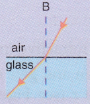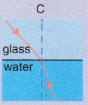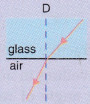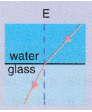Solution :
E.

Question 40: A vertical ray of light strikes the horizontal surface of some water :
(a) What is the angle of incidence ?
(b) What is the angle of refraction ?

Solution : (a) 00
(b) 00

Question 41: How is the reflection of light ray from a plane mirror different from the refraction of light ray as it enters a block of glass ?

Solution : The angle of reflection is equal to the angle of incidence but the angle of refraction is not equal to the angle of incidence.

Question 42: How does the light have to enter the glass :
(a) to produce a large amount of bending ?
(b) for no refraction to happen ?

Solution : (a) Obliquely; making a large angle of incidence.
(b) Perpendicular to the glass surface.

Question 43: (a) How can you bend light away from the normal ?
(b) How must light travel out of a substance if it is not going to be refracted ?

Solution : (a) By making the light enter from a denser medium to a rarer medium.
(b) Incidence should be at right angle to the surface of substance.

Question 44: Draw and complete the following diagrams to show what happens to the beams of light as they enter the glass block and then leave it :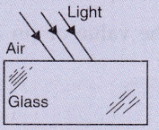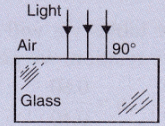Solution :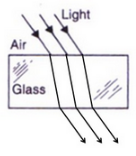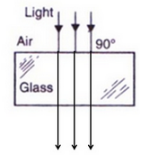Question 45: Why does a beam of light bend when it enters glass at an angle ? Why does it not bend if it enters the glass at right angles ?

Solution : A beam of light bends when it enters glass at an angle. This is due to refraction of light. It does not bend if it enters the glass at right angles because no refraction will occur in this case, the angle of incidence in this case is zero and angle of refraction is also zero.

Offer running on EduRev: Apply code STAYHOME200 to get INR 200 off on our premium plan EduRev Infinity!

61 docs

,

,

,

,

,

,

,

,

,

,

,

,

,

,

,

,

,

,

,

,

,

,

,

,

;